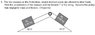# Two blocks on a double incline plane

• XwakeriderX

See attachment

F=MA
T=MA?

## The Attempt at a Solution

M1:
N1=M1gSin(32)
T=M1gCos(32)

Now does this work?:
T=MA
M1A=M1gCos(32)

and that would give me the acceleration for the X-axis and i could do the same for the Y-axis and get the magnitude?

#### Attachments

•2 blocks on incline.jpg
23.5 KB · Views: 2,100
Let the block M2 move down the plane and 'a' be its acceleration along the plane.
The forces acting on it are-
1)the normal force by the plane (perpendicular to the plane)
2)its weight vertically downwards
3)tension in the string

Forces perpendicular to the plane balance each other.
Forces along the plane are responsible for its acceleration.
No need to calculate separate components of acceleration for x and y axes respectively.
Just calculate its net acceleration along the plane.

Thank you very much!
Doing that ill set up T-Mgsin32=ma and ill do the same for m2 and solve for acceleration